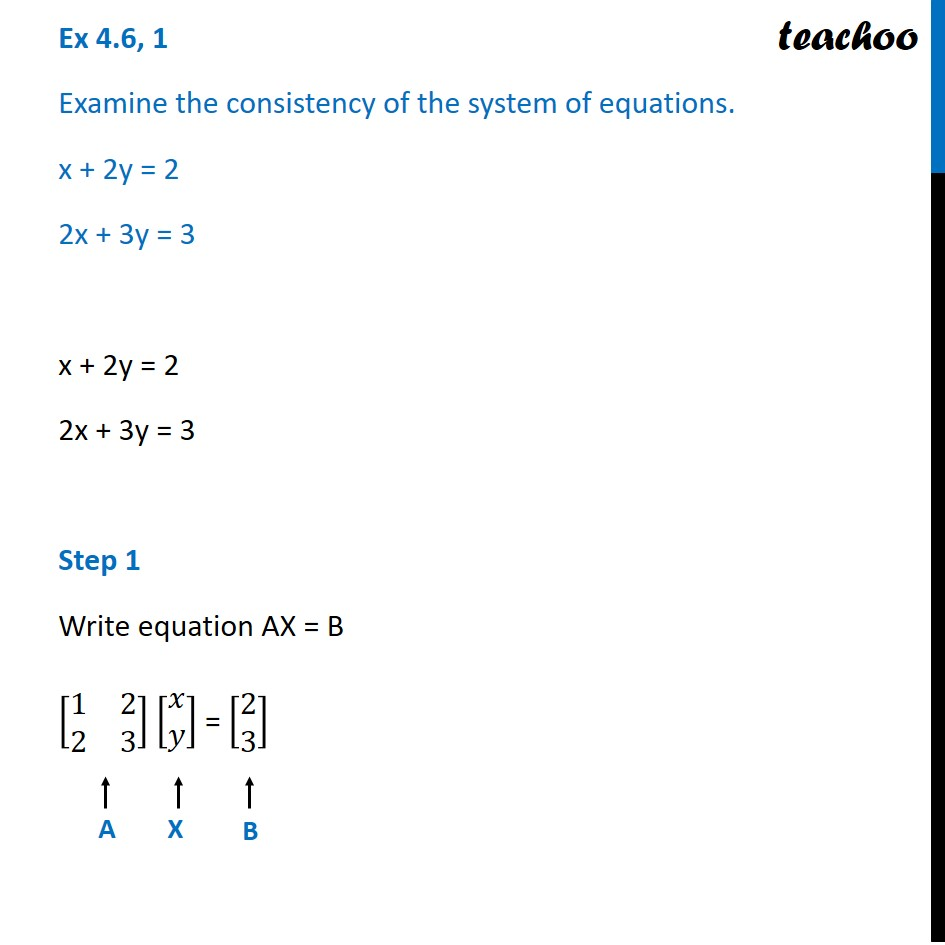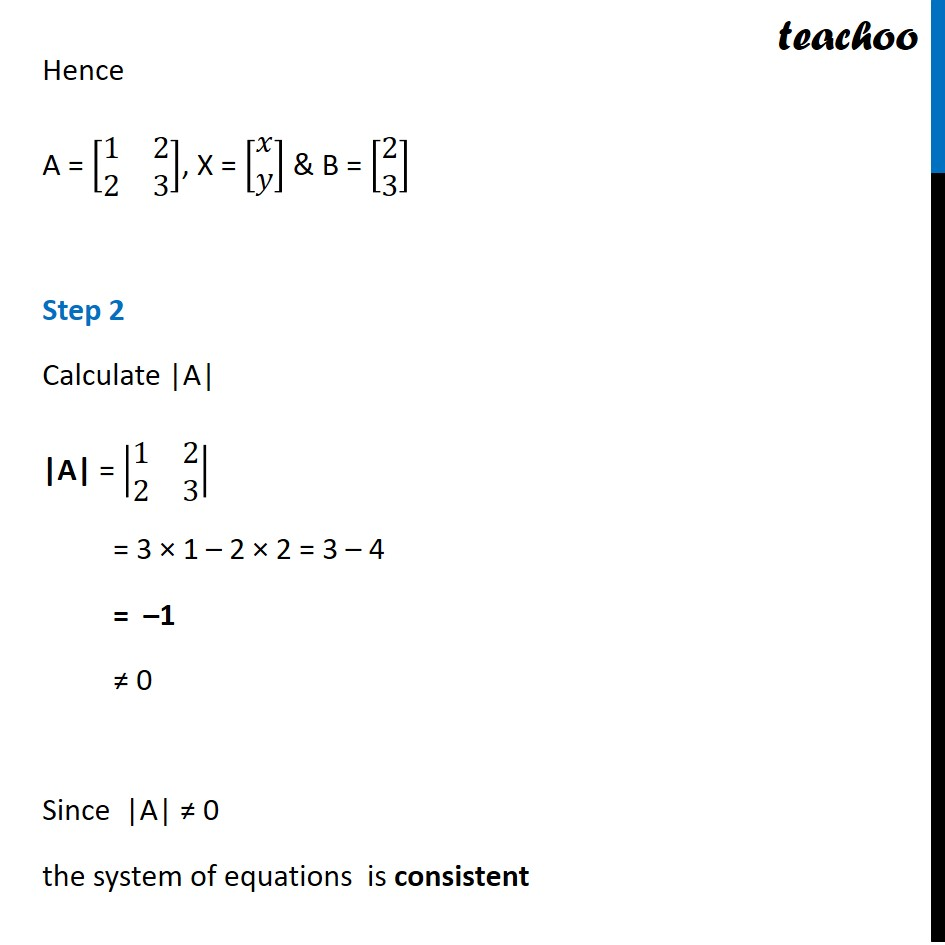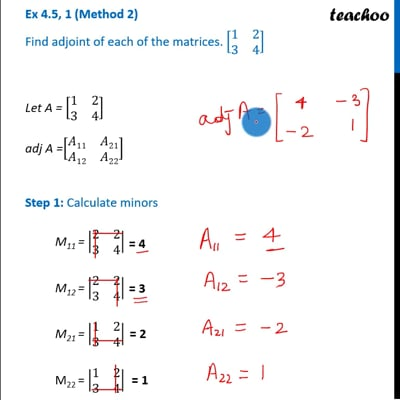Checking consistency of equations

Chapter 4 Class 12 Determinants
Concept wiseThis video is only available for Teachoo black users

Solve all your doubts with Teachoo Black (new monthly pack available now!)

### Transcript

Ex 4.6, 1 Examine the consistency of the system of equations. x + 2y = 2 2x + 3y = 3 x + 2y = 2 2x + 3y = 3 Step 1 Write equation AX = B [■8(1&2@2&3)] [■8(𝑥@𝑦)] = [■8(2@3)] Hence A = [■8(1&2@2&3)], X = [■8(𝑥@𝑦)] & B = [■8(2@3)] Step 2 Calculate |A| |A| = |■8(1&2@2&3)| = 3 × 1 – 2 × 2 = 3 – 4 = –1 ≠ 0 Since |A| ≠ 0 the system of equations is consistent